Courses

# Introduction to Structural Analysis and Equilibrium Civil Engineering (CE) Notes | EduRev

## Civil Engineering (CE) : Introduction to Structural Analysis and Equilibrium Civil Engineering (CE) Notes | EduRev

The document Introduction to Structural Analysis and Equilibrium Civil Engineering (CE) Notes | EduRev is a part of Civil Engineering (CE) category.
All you need of Civil Engineering (CE) at this link: Civil Engineering (CE)

Introduction

Structural Mechanics can be briefly described as the study of the behaviour of structures using the knowledge of mechanics. Such a description needs some understanding of the terms “structure” and “mechanics”. Structures include a wide variety of systems, such as buildings, bridges, dams, aircrafts, etc., that are built to serve some specific human needs (for example, habitation, transportation, storage, etc.). Students of Structural Mechanics should already have some basic knowledge of mechanics through the prerequisite courses of Engineering Mechanics (or Rigid-body Mechanics or Vector Mechanics) and Solid Mechanics (Mechanics of Deformable Solids or Mechanics of Materials). In Structural Mechanics, we apply our knowledge of the mechanics of rigid bodies and of deformable solids to the understanding of the behaviour of engineered structures. In Structural Mechanics, we mostly deal with mechanics of solids (i.e. deformable bodies). However, here we move on from studying the behaviour of structural members/materials (as in a course of Solid Mechanics) to studying the behaviour of real structures, or parts thereof. For example, instead of dealing with a beam or a column, we study how a building frame (Figure 1.1), composed of several beams and columns, behaves. In a similar way, we first learn about the loads that are applied to the whole structure, and not to individual members. Our knowledge of Structural Mechanics enables us to find the forces that act on individual members based on the loads that are acting on the whole structure. Stresses, strains, internal forces and deformations in members, then, can be obtained by using what we have already learned about the behaviour of deformable solids.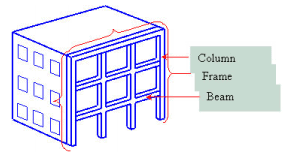Fig. 1.1 Frame in a building

Contents of this course of Structural Mechanics will focus on Civil Engineering structures only. Such structures are classified into various categories depending on the system/mode of classification:

(a) On the basis of its intended function/usage: Buildings, bridges, dams, industrial sheds, cable ways, chimneys, etc.(Figure1.2)

(b) On the basis of its form/load transfer mechanism: Beams, columns, floor slabs, arches, shells, trusses, frames,footings, etc.(Figure1.3)

(c) Considering the analysis perspective: 2-dimensional, 3-dimensional, determinate, indeterminate, etc.(Figure1.4)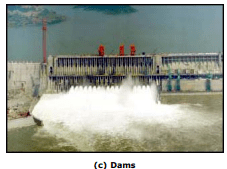Figure 1.2 Various types of structures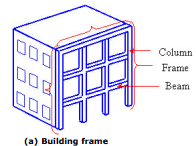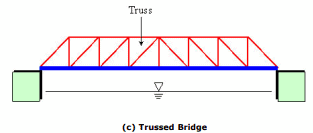Figure 1.3 Various structural forms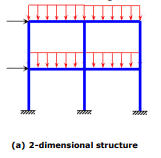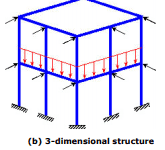Figure 1.4 2-dimensional and 3-dimensional model of structures

Similarly loads are also put into different categories, based on various criteria:

(b) Based on the direction of action: Gravity loads, lateral loads, etc. (Figure 1.5) a

(c) Based on time-variation: Static, dynamic, impulse, pseudo-static, etc. a

(d) Based on the mode of action/analysis point-of-view: Concentrated or point load, distributed load, moment, pressure, aaaaetc. (Figure 1.6).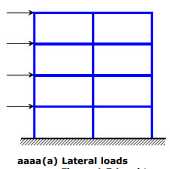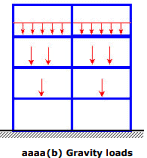Figure 1.5 Load types based on direction of action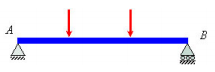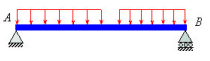(a) Concentrated or point loads on         (b) Distributed loads on a simply

a simply supported beam                                supported beam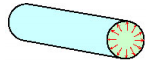(c) Pressure acting on inner surface of a cylinder

Figure 1.6 Load types based on analysis point of view

Equilibrium

The concept of equilibrium is the most central one in the subject of Statics. When the net effect or the resultant of all the forces (and couples) acting on a system is zero, the system is said to be in equilibrium. Thus, based on the resultant of all the forces , and the resultant of all the moments (couples) , the vector equations of equilibrium are

R= ∑F=0 and M= ∑M=0                        (1.1)

The two vector equations of equilibrium can be expressed alternatively as scalar equations of equilibrium for a system of forces in 3 dimensions ( x , y & z ), as

∑Fx =0, ∑Fy =0, ∑Fx =0         (1.2)

∑Mx =0, ∑My =0,∑Mz =0          (1.3)

Here,  ∑Fx represents the algebraic summation of components of all the forces in x-direction. This summation is same as the resultant (net effect) of all the forces in x-direction.

This set of six equilibrium equations can be narrowed down to three scalar equations in case of a planer force system (forces acting in two dimensions only)

∑ Fx=0, ∑Fy=0, ∑Mz=0              (1.4)

Figures 1.7 & 1.8 illustrate how resultants are obtained for a two-dimensional (planer) force system.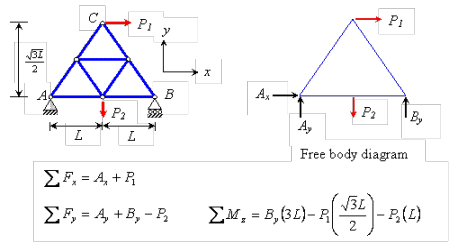Figure. 1.7 Obtaining resultants for a truss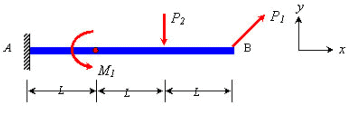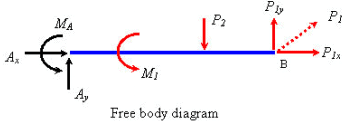∑Fx=Ax+P1x

∑Fy=Ay+P1y-P2

∑Mz= -Ay(3L)+MA+P2(L)+M1

Figure 1.8 Obtaining resultants for a cantilever system

These equations provide the necessary and sufficient forces to keep a system in equilibrium. The omission of a force that is acting on a system or the inclusion of a force that is not acting on the system produces erroneous results in analyzing the behaviour of the system. Hence, it is of utmost importance to understand exactly what the mechanical system under consideration is and the forces that are acting on the specific system. A system is a body or a combination of connected bodies. The bodies can be either rigid or deformable (even fluids can be treated as body). For Structural Mechanics, we will restrict ourselves to the study of rigid and deformable solids only. For the important task of identifying the forces (and couples) acting on a system, we take the help of Free Body Diagrams. Thus, drawing a free body diagram becomes the first and foremost task in solution of problems in mechanics.

The free body diagram of a body (or its part, or a connected system of bodies) is obtained by isolating it from the all other surrounding bodies. The diagram detaches the system in consideration from all mechanical contacts with other bodies and sets it free . The other bodies are not shown in the diagram, but they are replaced by the forces (and couples) that they apply on the system for which we are drawing a free body diagram. The following examples show how to obtain the free body diagram for a system and also the equilibrium equations for the same system.

Offer running on EduRev: Apply code STAYHOME200 to get INR 200 off on our premium plan EduRev Infinity!

,

,

,

,

,

,

,

,

,

,

,

,

,

,

,

,

,

,

,

,

,

;